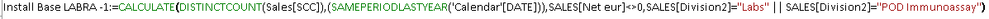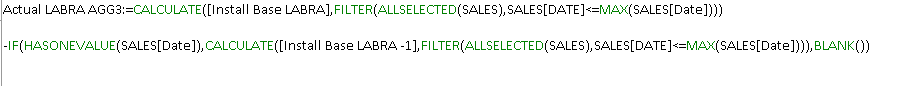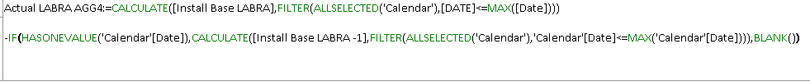cancel
Showing results for
Did you mean:Helper II

## Aggregated Running total for YoY/Change

Hi Everyone,

I have an Install base (customer count) measure which distinctcounts the number of customer IDs as follows:I also have a similiar measure for the same period last year:I use the two together to create a measure which reflects the change in customer count:I'm trying to create the same measure but Aggregated but I'm struggling with this and getting contiguous date selections dax-Or when i try it this way i get too high numbers:So to try to recreate what I have on the right end below, summing the months as a running/aggregate total.Thank you everyone,

Eyal

1 ACCEPTED SOLUTIONSuper User

Hi:

When you do your "Same Period LY measure, can you do it like this:

SPLY Install Base LABRA = CALCULATE([Install Base LABRA], SAMEPERIODLASTYEAR(Dates[Date]))
Then subtract your initial measure from this. I'll call it VAR Install Base LABRA

To get cumlative total for the VAR Install Base LABRA

= CALCULATE([VAR Install Base LABRA], FILTER(ALLSELECTED(Dates),
Dates[Date] <= MAX(Dates[Date])))

Normally this should work. Can you give it a try? Thanks
5 REPLIES 5Helper II

Thanks @Whitewater100 , i'll try changing to my calendar table (i used the detail table date instead) and will update.Super User

Hello:

Great. I hope that works for you and if it does can you mark as solution? Thanks...Super User

Hi:

I think you may be gettting that message as you may not have a date table, with continuous dates starting before your data starts and ending at end of ear after. When you mark it as a date table and connect it on the one side to your many-side tables, then the built-in time intel functions work great.

I can paste some date table DAX code that you can use as a Date Table if you want to.

Dates =

-- Specify a start date and end date

VAR StartDate = Date(2010,1,1)

VAR EndDate = Today()

VAR FiscalMonthEnd = 6

-- Generate a base table of dates

VAR BaseTable = Calendar(StartDate, EndDate)

-- Add the Year for each individual date

-- Add the calendar month and other month related data for each date

Years,

"Month",MONTH([Date]),

"Year and Month Number",FORMAT([Date],"YYYY-MM"),

"Year and Month Name",FORMAT([Date],"YYYY-MMM"),

"Fiscal Year", IF( FiscalMonthEnd = 12, YEAR([Date]), IF( MONTH([DATE]) <= FiscalMonthEnd, YEAR([DATE])-1, YEAR([Date]))),

"Fiscal Month", IF( FiscalMonthEnd = 12, MONTH([Date]),

IF( MONTH([Date]) <= FiscalMonthEnd, FiscalMonthEnd + MONTH([Date]), MONTH([Date]) - FiscalMonthEnd))

)

-- Add the Quarter and other quarter related data for each date

Months,

"Quarter",ROUNDUP(MONTH([Date])/3,0),

"Year and Quarter",[Year] & "-Q" & ROUNDUP(MONTH([Date])/3,0))

-- Add the Day and other day related data for each date

Quarters,

"Day",DAY([Date]),

"Day Name",FORMAT([Date],"DDDD"),

"Day Of Week",WEEKDAY([Date]),

"Day Of Year", DATEDIFF (DATE(YEAR([Date]),1,1), [Date], DAY) + 1)

-- Add the Week (assuming each week starts on a Sunday) and other week related data for each date

Days,

"Week Of Month (Sunday)",INT((DAY([Date])-1)/7)+1,

"Week of Year (Sunday)",WEEKNUM([Date],1),

"Year and Week (Sunday)",[Year] & "-W" & WEEKNUM([Date],1))

-- Add an 'Is Working Day' column which will be true for all days but Saturday and Sunday.

Weeks,

"Is Working Day", NOT WEEKDAY( [Date] ) IN {1,7})

RETURN WorkingDays

I hope this helps!Helper II

Hi @Whitewater100 I tried switiching as follows to my calendar table:But I"m still not getting what I need to get (red arrow to yellow)I'm getting the blue..Super User

Hi:

When you do your "Same Period LY measure, can you do it like this:

SPLY Install Base LABRA = CALCULATE([Install Base LABRA], SAMEPERIODLASTYEAR(Dates[Date]))
Then subtract your initial measure from this. I'll call it VAR Install Base LABRA

To get cumlative total for the VAR Install Base LABRA

= CALCULATE([VAR Install Base LABRA], FILTER(ALLSELECTED(Dates),
Dates[Date] <= MAX(Dates[Date])))

Normally this should work. Can you give it a try? Thanks